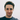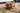David Morton

# Deterministic vs. Pure functionsPhoto by Michael Oxendine

All pure functions are deterministic, but not all deterministic functions are pure. Pure functions are easier to reason about and test, you should aim to implement pure functions.

Pure functions are deterministic AND they have no side effects. Deterministic functions may have side effects. Both types of functions return the same output every time they are invoked with the same inputs between invocations.

You should aim to implement pure functions, they are easier to reason about and test.

## Deterministic functions

In mathematics, computer science and physics, a deterministic system is a system in which no randomness is involved in the development of future states of the system. A deterministic model will thus always produce the same output from a given starting condition or initial state.

https://en.wikipedia.org/wiki/Deterministic_system

Deterministic functions will produce the same results given the same inputs. If invoked multiple times using the same input value, the output value will remain the same.

``````let variableX = 0;
const deterministicFunction = (a, b) => {
variableX += a + b // side effect
return a + b
}
deterministicFunction(5, 7) // 12``````

This deterministic function will return `12` for inputs `5` and `7` no matter how many times we invoke it. It will also increment `variableX` outside of the functions scope, which is known as a side effect. This is a deterministic function, but its not pure because it generates a side effect by updating `variableX`.

Some other examples of side effects are:

• Modifying variables outside of the functions scope
• `console.log()`
• HTTP requests `fetch()`
• Querying the DOM `document.*()`
• Writing to the file system `fs.writeFile()`
• Modifying input variables

If your function is involved in a side effect it may be deterministic, but its definitely not pure.

## Pure functions

In computer programming, a pure function is a function that has the following properties:

• Its return value is the same for the same arguments (no variation with local static variables, non-local variables, mutable reference arguments or input streams from I/O devices).
• Its evaluation has no side effects (no mutation of local static variables, non-local variables, mutable reference arguments or I/O streams).

https://en.wikipedia.org/wiki/Pure_function

``````const pureFunction = (a, b) => {
return a + b
}
pureFunction(5, 7) // 12``````

This deterministic function will return `12` for inputs `5` and `7` no matter how many times we invoke it, and you’ll notice that it does not generate any side effects when invoked. This is known as a pure function.

Another way to think about pure functions is that they can be replaced by the value the function produces, without affecting the overall programs behaviour. This is known as Referential transparency and is an attribute of pure functions.

## Nondeterministic functions

``````let variableX = 0;
const hardToTestFunction = (a, b) => {
return a + b * variableX // relies on state outside of function scope
}
hardToTestFunction(5, 7) // 89
hardToTestFunction(5, 7) // 173``````

When the result of a function is not strictly determined by the inputs, it is nondeterministic, and therefore not pure. In the example above, the result returned by the function is affected by the value of `variableX` and this will change with each new invocation. The value in `variableX` could also be changed by another part of the system, which may change the returned result.

## Testing

Let’s take a look at testing the functions in the above examples, we’ll start off with `nondeterministic`, then `deterministic`, and finally the `pure` function.

### Nondeterministic

``````let variableX = 0;
const hardToTestFunction = (a, b) => {
return a + b * variableX // relies on state outside of function scope
}

// test
variableX = 0 // given
let actual = hardToTestFunction(5, 7); // when
console.assert(actual === 89, actual) // then``````

Nondeterministic functions are difficult to reason about and test effectively, stay away from these or refactor them if you can. In the above example the results we are asserting could be affected by another part of the system changing the value of `variableX`. We do not know how or when this could happen, so how do we test this effectively?

### Deterministic

``````let variableX = 0;
const deterministicFunction = (a, b) => {
variableX += a + b // side effect
return a + b
}

// test
let actual = deterministicFunction(5, 7); // when
console.assert(actual === 12, actual) // then``````

Deterministic functions are a little easier to test than nondeterministic functions because we know that we will get the same result each time we call this function provided we do not change the inputs.

But when testing, do we need to also test the change in the overall system behaviour as a result of invoking this function? How do we scope this test? How do we know we have tested all related system changes?

Function side effects usually require mocking or spying on external dependencies, we should try to avoid this as much as possible.

### Pure

``````let variableX = 0;
const pureFunction = (a, b) => {
return a + b
}

// test
let actual = pureFunction(5, 7); // when
console.assert(actual === 12, actual) // then``````

Pure functions are easy to reason about and test. The results of invoking these functions are deterministic, and we don’t have to worry about the overall systems behaviour being changed. Our tests can focus on the logic implemented in the pure function itself, and there is no need to mock or spy on external dependencies.

The majority of our code should live inside pure functions.

## Conclusion

I’ve discussed the differences between deterministic, pure, and nondeterministic functions in this post. The examples provided are simplistic to illustrate some important points, and I understand that your production code may not be as easy to categorise.

Somewhere in your program you may need to interact with the file system, and make some network requests, and query the DOM. This functionality should be kept separate from your core functionality or business logic. We can create shells or wrappers to orchestrate and integrate the different parts of our system, while keeping our core code pure and clean.

Practicing test driven development teaches you to write code that is easier to reason about and maintain. If you like the idea of pure functions and you are not sure how to apply what you have learned here try introduce some TDD into your practice. You’ll spend less time debugging your code and will get organisation and more pure functions for free!.

## References